×

Search anything:

# Firoozbakht’s conjecture

#### List of Mathematical Algorithms AlgorithmsFREE BOOK -> Problems for the day before your Coding Interview (on Amazon)

In this article, we examine the relationship between Farideh Firoozbakht's famous conjecture about prime numbers, which states that the sequence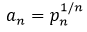where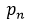is the nth prime number, is strictly decreasing, and the prime gap function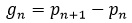which is the difference between the nth and (n + 1)th prime numbers. Furthermore, Firoozbakht's conjecture is linked to upper limits for the prime gap function in terms of n. Asymptotic outcomes for both the conjecture and the function help us understand the interrelationships.

Farideh Firoozbakht's initial conjecture in 1982 was that the sequence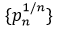is strictly decreasing, i.e.,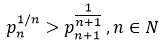and the first few values of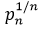are shown below: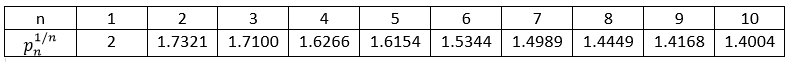and the speculation has been confirmed for all primes less than 4x(10)^18.

# Related forms of Firoozbakht’s conjecture

Following are some alternative formulations of the hypothesis. Here n∈N.

•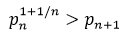(1),
•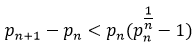(2),
•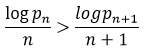(3),
•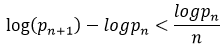(4)

These are easily provable by direct analysis and are by no means a complete list. The left hand side of (2), however, is the nth prime gap, and if the conjecture is correct,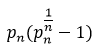is an upper bound in terms of n for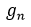.

In fact, if (1) is accurate, an upper bound ofis found to be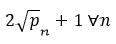. (1) may also be used to improve on the Baker-Harman-Pintz's upper bound of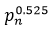for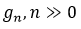. More conjectural upper limits forin terms of n will be showed, but for the time being, we will focus on certain known findings that give an approach to demonstrating (1).

# Evidence to back up the conjecture

## Proving (1) for infinitely many n∈N:

Zhang demonstrated that,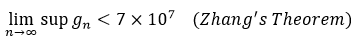Outcome: There exists an infinite number of n∈N such that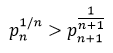Proof: We assume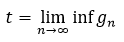and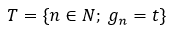Then, according to Zhang's Theorem, T is infinite and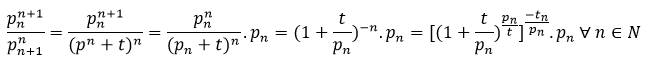Now,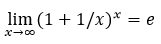Specifically, for any sufficiently big n∈T,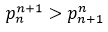. This is identical to the statement of the theorem. Thus,
Zhang’s Theorem ⇒ (1) applies for infinitely many n ∈ N

## Proving (1) for almost all n ∈ N:

In order to prove this statement, we will require the following two well-known theorems.
Theorem 1: Let us consider the set of the first n−1 differences between consecutive primes, i.e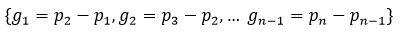. Let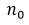be the number of differences in this set such that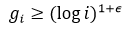where ϵ > 0 is fixed. We then have the limit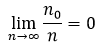. Therefore, if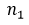is the number of differences in this set such that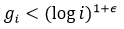we have that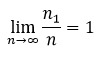. That is, practically all of the set's differences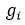fulfil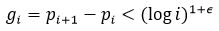Theorem 2: The following asymptotic formula holds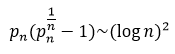Proof: We have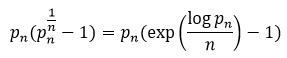. The prime number theorem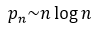, consequently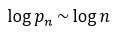, derives directly from the formula exp(x)=1+x+o(x)(x→0). The theorem is proved.
Outcome: The inequalities in (1) to (4) hold for practically all successive prime pairings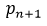and.

Proof: According to Theorem 2, since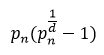is asymptotically comparable to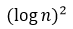, there must be some value of n∈N, such that ∀j>n, j∈N,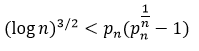.
Furthermore, theorem 1 with ϵ=12 shows that for nearly all pairs of successive primes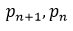, the inequality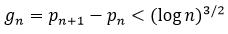holds for almost all pairings of consecutive primes. This is inequality (2), and because (2) and (1) are similar, (1) holds true for almost all n∈N.

# Upper boundaries of the prime gap function

The prime gap function,, is inextricably linked to Firoozbakht's conjecture. There are a few speculative upper bounds on.
It is proved that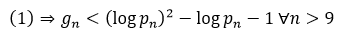It can also be proven that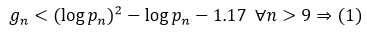Following the demonstration of the previous implication, we shall show how (1) follows from the assumed reality of another such conjectural upper bound, one of a family of such bounds.
Outcome: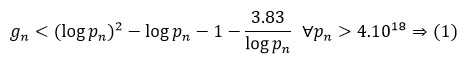Proof: We notice that (1) has been proven for all primes less than 4x(10)^18, which is why we include it in the stated condition.
Using the Panaitopol formula for π(x), we get an upper bound for the prime-counting function π(x), which is provided by: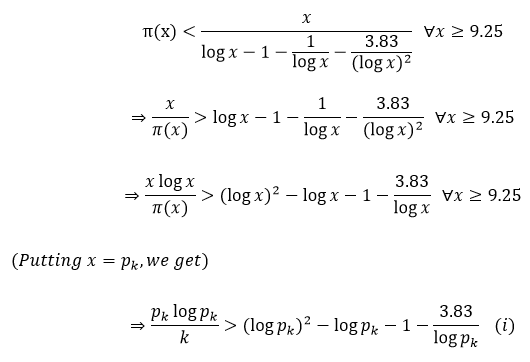We are not including our x ≥ 9.25 constraint since we are examining primes higher than 4x(10)^18, and primes up to the limit have already been proven to obey the conjecture. As a result, the primes in question are large enough.
We know using calculus,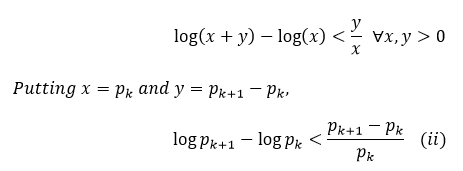Thus we get from the given inequality,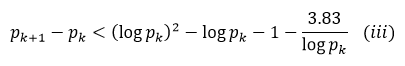From (i), (ii) and (iii), we get,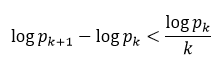which is (4), one of the equivalent forms of (1). As a result, we have demonstrated that if the higher bound indicated above holds, then (1) is true.
There are two additional conjectured upper boundaries for, which are as follows: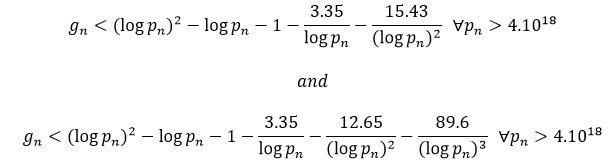and the truth of either of these two implies (1).

# Program to verify the conjecture

Following is a C++ Program to verify the conjecture:

``````#include <bits/stdc++.h>
using namespace std;

void firoozbakhtConjecture(int n)
{
bool prime[n + 1];
memset(prime, true, sizeof(prime));
for (int p = 2; p * p <= n; p++)
{
if (prime[p] == true)
{
for (int i = p * p; i <= n; i += p)
prime[i] = false;
}
}
double i=1;
for (int p = 2; p <= n; p++)
if (prime[p])
{
cout << double( pow(p,(1/i)) ) <<"\n";
i++;
}
}

int main()
{
int n = 100;
firoozbakhtConjecture(n);
return 0;
}
``````

The above C++ program will yield the following output: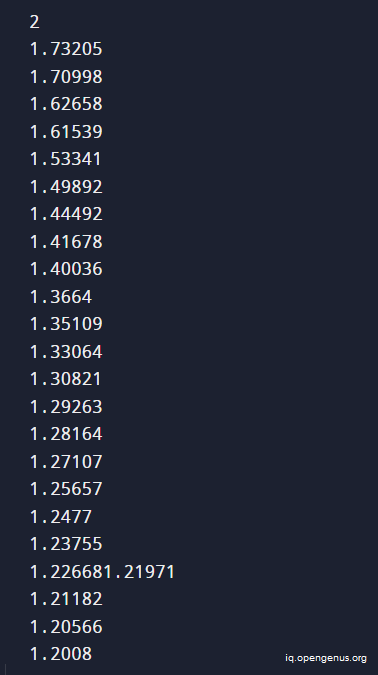The series keeps on decreasing for a large range and hence the conjecture can be proved till that given range. You can run it continuously till two consequtive elements increase (which will disprove the conjecture).

These techniques are used to verify conjectures till a given limit.

# Associated conjectures

Three more conjectures were proposed in relation to the Firoozbakht Conjecture.
The Nicholson Conjecture-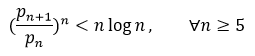Forgues' Conjecture-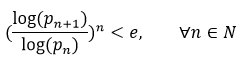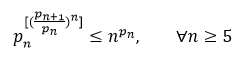To see how these conjectures connect to the Firoozbakht Conjecture, consider the following inequalities:
Theorem 1: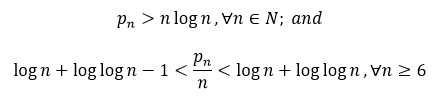This inequality is know as the Rosser’s Theorem.

Theorem 2: Farhadian ⇒ Nicholson ⇒ Firoozbakht ⇒ Forgues
Proof Assume the Farhadian Conjecture is correct. Thereafter, using logarithms,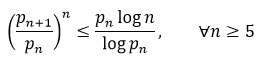From previous theorem,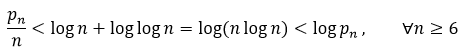This suggests the Nicholson Conjecture. Assuming the Nicholson Conjecture, Rosser's Theorem states that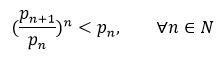which is the same as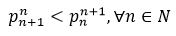This corresponds to the Firoozbakht Conjecture. Taking logarithms out from previous inequality, we get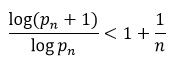When we increase to the n-power, we obtain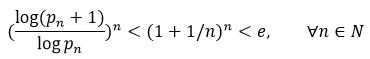which is the Forgues Conjecture.

The Firoozbakht conjecture is one of the most powerful upper limits on prime gaps, rivalling the Cramér and Shanks conjectures. Cramér predicted that the gaps around p are no larger than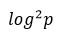, while Shanks proposed the asymptotic equivalence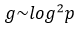for maximal prime gaps g.#### Harshita Singh

Harshita Singh is pursuing her B. Tech in CSE from Harcourt Butler Technical University, Kanpur (2023). She is an Intern at OpenGenus.

Firoozbakht’s conjecture